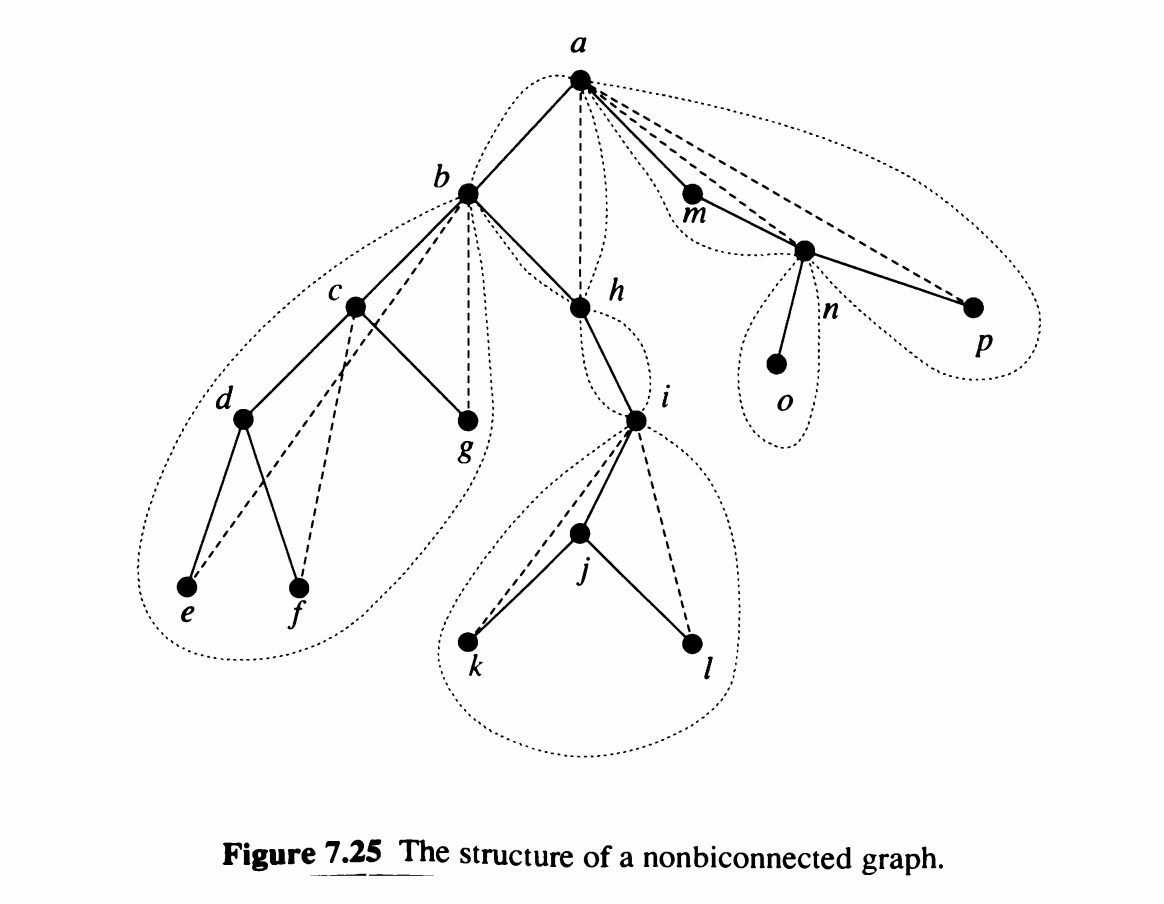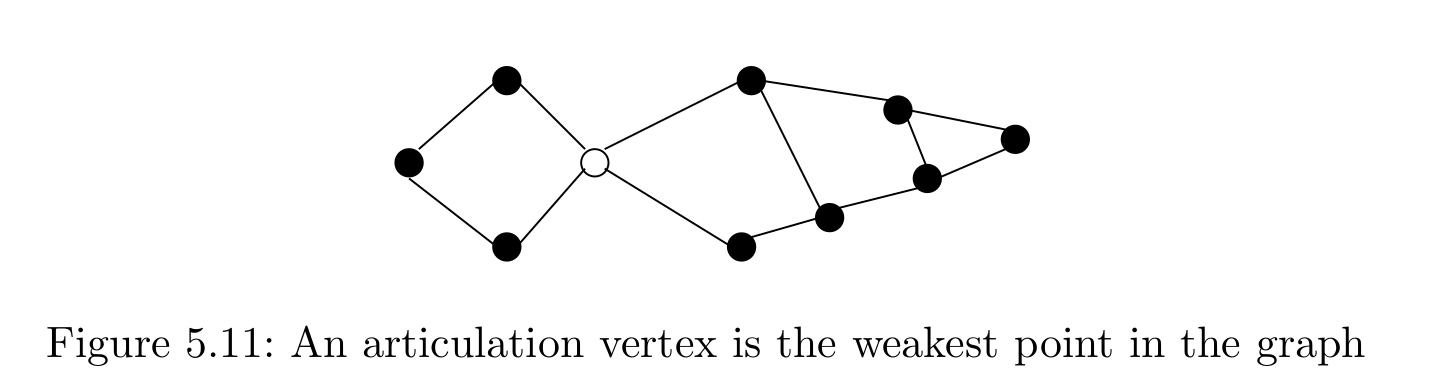## § Articulation points

I find DFS fascinating, and honestly insane for how much structral information of the graph it manages to retain.
A vertex $v$ is an articulation point of a graph $G$ if the removal of $v$ disconnects the induced subgraph.

#### § Tactic 1 - Inductively

We first solve the super easy case with the root, and then try to see if we can treat other cases like the root node case, then we're good. Here, we are given an graph $G$, and we are thinking about a DFS tree $T_G$ of the graph $G$.

#### § Thinking about the root

When is the root an articulation point? If the root has multiple children, then it is an articulation point; If we remove the root, then it disconnects the children. This is because we have an undirected graph, where we only have back edges, and no cross edges. A back edge can only go from a node to its ancestor. If we remove the root, the back edges cannot save us, for there is no ancestor higher than the root to act as an alternate path

#### § Non root vertex

When is a non root vertex $v$ an articulation point? When there is some child $w$ of $v$ such that the subtree of $w$ cannot escape the subtree of $v$. That is, all back edges from $w$ do not go above $v$. If we were to now remove $v$, then $w$ would be disconnected from the rest of the graph.
Alternate phrasing: When all cycles in the subtree of $w$ are within the subtree of $v$. This means that the backedges cannot go above $v$. If $w$ could build a cycle that goes above $v$, then $v$ would not be an articulation point, because it'll be involved in some cycle $v \mapsto w \mapsto \mapsto p \mapsto v$, which gives us an alternative path to reach $w$ even if $v$ is removed.
One way to imagine this maybe to imagine $v$ as the new root, and the other stuff that's above $v$ to be to the left of $w$. That way, if we could go to $w$, we get a cross edge from the "new root"(v) and the "other section" (the part that's connected by a cross edge). If we prevent the existence of these "fake cross edges", we're golden, and $v$ is then an articulation point.

#### § Tactic 2 - Structurally / Characterization

Next we follow a "mathematical" development, where we build theorems to characterize k-connectedness and use this to guide our algorithm design

#### § Menger's theorem

Let $G$ be a connected undirected graph. Let $u, v$ be two non-adjacent vertices. The minimum number of vertices whose removal from $G$ disconnects $u$ and $v$ is equal to the maximal number of vertex disjoint paths from $v$ to $u$.

#### § Whitney's theorem (corollary)

An undirected graph is $k$ connected iff $k$ vertices must be removed to disconnect the graph.

#### § Biconnected components

Menger's theorem tells us that a graph is not biconnected iff we can find a vertex whose removal disconnected the graph. Such a vertex is an articulation vertex.
A biconnected component is a maximal subset of edges, such that the induced subgraph is biconnected. Vertices can belong to many components; Indeeed, articulation vertices are those that belong to more than one component.#### § Lemma: Characterization of biconnected components

Two edges belong to the same biconnected component iff there is a cycle containing both of them. [This lemma is silent about biconnected components of single edges ]
We show that a cycle is always contained in a single binconnected component. If a cycle contains edges from more than one biconnected component, then we can "fuse" the biconnected components together into a single, larger, biconnected component.

#### § Tactic 3 - 'Intuitively'

We look at pictures and try to figure out how to do this.

#### § DFS for articulation vertices - undirected:• The connectivity of a graph is the smallest number of vertices that need to be deleted to disconnect the graph.
• If the graph has an articulation vertex, the connectivity is 1. More robust graphs that don't have a single point of failure/articulation vertex are said to be binconnected .
• To test for an articulation vertex by brute force, delete each vertex, and check if the graph has disconnected into components. this is $O(V(V+E))$ time.
Joke: an articulate vertex is one that speaks very well, and is thus important to the functioning of the graph. If it is killed, it will disconnect society, as there is no one to fulfil its ability to cross barriers with its eloquent speech.

#### § Articulation vertices on the DFS tree - undirected

• If we think of only the DFS tree for a moment of an undirected graph and ignore all other edges, then all interneal non-leaf vertices become articulation vertices, because they disconnect the graph into two parts: the part below them (for concreteness, think of a child leaf), and the root component.
• Blowing up a leaf has no effect, since it does not connect two components , a leaf only connects itself to the main tree.
• The root of the tree is special; If it has only one child, then it acts like a leaf, since the root connects itself to the only component. On the other hand, if there are multiple components, then the root acts like an internal node, holding these different components together, making the root an articulation vertex.

#### § Articulation vertices on the DFS graph - undireced

• DFS of a general undirected graph also contains back edges . These act as security cables that link a vertex back to its ancestor. The security cable from x to y ensures that none of the nodes on the path [x..y]can be articulation vertices.
• So, to find articulation vertices, we need to see how far back the security cables go.
int anc[V]; int dfs_outdeg[V];
void processs_vertex_early(int v) { anc[v] = v; }
void process_edge(int x, int y) {
if (dfsedge[x][y].type == TREE) { dfs_outdeg[x]++; }
// y <-*
//     |
//     BACK
//     |
// x --*
if (dfsedge[x][y].type == BACK && (parent[y] != x)) {
if(entry_time[y] < entry_time[anc[x]]) {
anc[x] = y;
}
}
}In a DFS tree, a vertex v (other than the root) is an articulation vertex iff v is not a leaf and some subtree of v has no back edge incident until a proper ancestor of v.